Question

(1 point) Use implicit differentiation to find an equation of the tangent line to the curve 2xy3+xy=302xy3+xy=30 at the point (10,1)(10,1).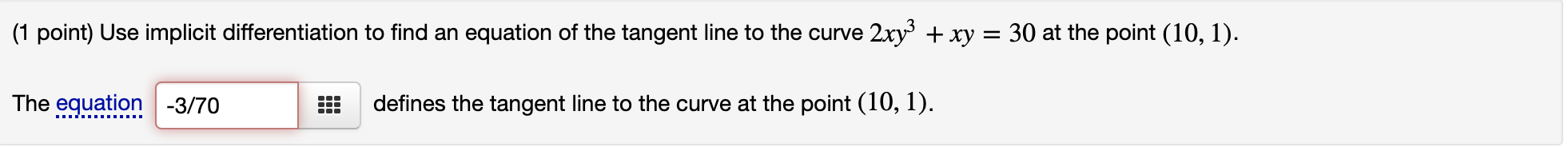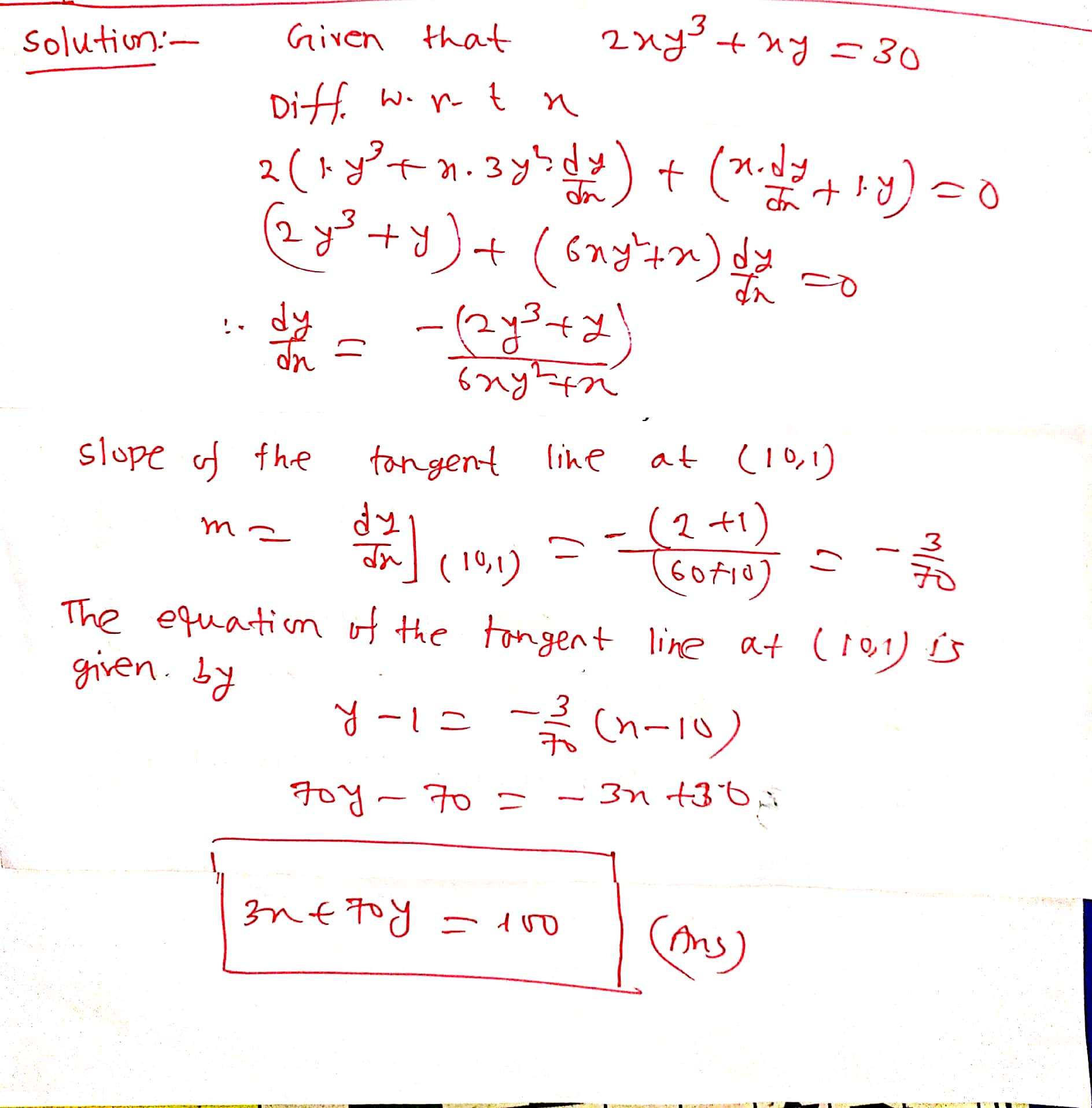#### Earn Coins

Coins can be redeemed for fabulous gifts.

Similar Homework Help Questions
• ### Use implicit differentiation to find the equation of the tangent line to the curve xy^3+xy=5 at the point (4,1)

Use implicit differentiation to find the equation of the tangent line to the curve xy^3+xy=5 at the point (4,1) . The equation of this tangent line can be written in the form y = mx+b where m is:? and where b is:?

• ### Use implicit differentiation to find the equation of the tangent line to the curve xy^3+2xy=9 at the point (31)

Use implicit differentiation to find the equation of the tangent line to the curve xy^3+2xy=9 at the point (31). The equation of this tangent line can be written in the form y=mx+b where m is

• ### Use implicit differentiation to find the equation of the tangent line to the curve xy3+xy=14 at the point (7,1)

Use implicit differentiation to find the equation of the tangent line to the curve xy3+xy=14 at the point (7,1) . The equation of this tangent line can be written in the form y=mx+bi dont seem to no how to find m or b

• ### (1 point) Use implicit differentiation to find the slope of the tangent line to the curve...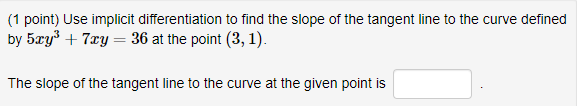(1 point) Use implicit differentiation to find the slope of the tangent line to the curve defined by 5xy + 7xy = 36 at the point (3,1). The slope of the tangent line to the curve at the given point is

• ### Use implicit differentiation to find the equation of the tangent line at the given point. z?...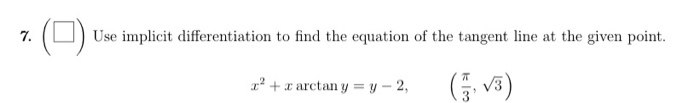Use implicit differentiation to find the equation of the tangent line at the given point. z? + x arctan y = y -2,

• ### Use implicit differentiation to find an equation of the tangent line to the graph of the...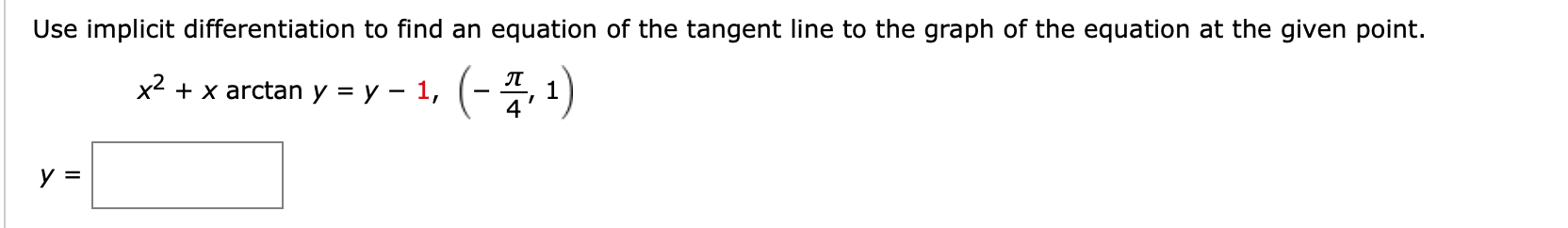Use implicit differentiation to find an equation of the tangent line to the graph of the equation at the given point. x2 + x arctan y = y -

• ### Find the equation of the tangent line to the curve at the given point using implicit...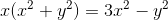Find the equation of the tangent line to the curve at the given point using implicit differentiation. Remember: equation of a line can be found by y-y1=m(x-x1) where m is the slope of the line and (x1,y1) is any point on the line. Curve: at (1,1)

• ### 3. Use implicit differentiation for the curve x3 + 3y4 = xy and then find the...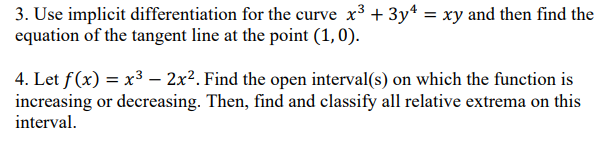3. Use implicit differentiation for the curve x3 + 3y4 = xy and then find the equation of the tangent line at the point (1,0). 4. Let f(x) = x3 – 2x2. Find the open interval(s) on which the function is increasing or decreasing. Then, find and classify all relative extrema on this interval

• ### Use implicit differentiation to find the slope of the tangent line to the curve 5x^3 y^2...

Use implicit differentiation to find the slope of the tangent line to the curve 5x^3 y^2 - 4x^2 y = 1at the point (1,1) m=

• ### Implicit Differentiation

Use implicit differentiation to find an equation of the tangent line to the curve at the given point.9x2 + xy + 9y2 = 19, (1, 1) (ellipse)